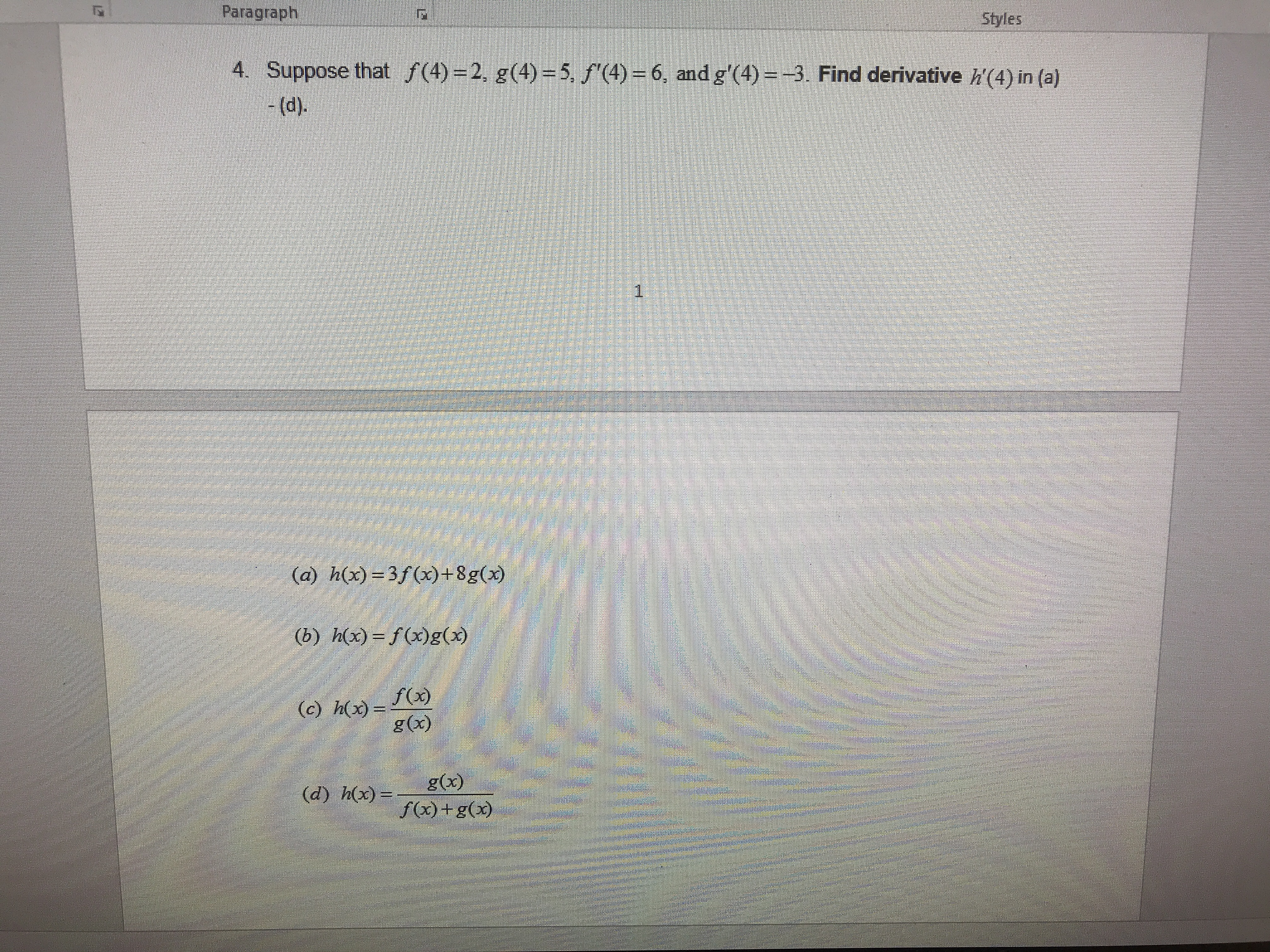# ParagraphStyles4. Suppose that f(4)-2, g(4)-5, /(4-6, and g'(4)- 3. Find derivative h'(4) in (a)(a h(x) 3f +8g)(b) ho)-g)f(x)g (x)g(x)f)+g)

Question
90 viewshelp_outlineImage TranscriptioncloseParagraph Styles 4. Suppose that f(4)-2, g(4)-5, /(4-6, and g'(4)- 3. Find derivative h'(4) in (a) (a h(x) 3f +8g) (b) ho)-g) f(x) g (x) g(x) f)+g) fullscreen
check_circle

Step 1

(a)

Differentiate h(x) = 3f (x) +8g (x) with respective to x as .

Substitute x = 4 in h’(x) to obtain the value of h’(4).

Step 2

(b)

Differentiate h(x) = f (x) g (x) with respective to x as .

Substitute x = 4 in h’(x) to obtain the value of h’(4).

Step 3

(c)

Differentiate h(x) = f (x) / g (x) with respective to ...

### Want to see the full answer?

See Solution

#### Want to see this answer and more?

Solutions are written by subject experts who are available 24/7. Questions are typically answered within 1 hour.*

See Solution
*Response times may vary by subject and question.
Tagged in

### Calculus